# An Alternative To The Sharpe Ratio

## Applications of Time Series Analysis (ARMA-GARCH) To Improve Risk-Adjusted Return Screening Procedures.

You have been given the daily returns of several strategies / funds over the past year, how would you determine which one provides the best investment opportunity given only this information?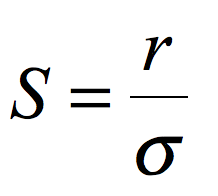The Sharpe Ratio (r = average daily return, sigma = standard deviation in daily returns). Some of you may immediately jump and say that I’ve forgotten to subtract the risk-free rate from the strategy returns, but these days it's so close to zero we can forget about it.

In theory this should be an excellent metric to solve our original problem, we can now declare the strategy with the best Sharpe Ratio as the best investment opportunity.

Hence if both r and sigma are random variables the Sharpe ratio itself must also carry some uncertainty in its true value.

How confident can we be that one strategy is truly superior to the other or that either strategy will have a positive return in the long run?

In short if the confidence intervals of two strategies Sharpe ratios overlap then we cannot be confident that the future performance of strategy A will be significantly better than strategy B. Similarly if either strategy’s confidence interval contains zero, we cannot even be confident that in the long run we will make any money!

## But what about all those assumptions you made earlier that you said were not true!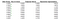You can see that the sum of returns approximates the true compound return, but the application of Taylor series approximation twice (as in the “Sum of Returns” column) is worse than only applying the approximation once (See “Exponential Approximation”)

## What is an ARMA-GARCH Model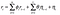ARMA(u,v) Process — Yes its as simple as it looks, the complicated bit is actually estimating the theta’s and phi’s but R will take care of that for you. Think of this just like a linear regression — but on the past randomness and values of the series rather than on other variables.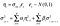GARCH(p,q) Model, the square of the volatility (variance) of the random terms are modeled as fluctuating and thus rescaling the white noise epsilon_t’s variance. Again think of the conditional variance as a regression on past shocks to the series and past conditional variances. Again RStudio (R; fGarch Library) will take care of estimating the alphas and betas.

# Estimating Intrinsic Returns of 500 Randomly Selected Mutual Funds

## Sharpe vs Intrinsic as Predictor of Future Returns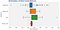Box plot of Post COVID (Out of Sample) Returns on 500 Mutual Funds, grouped by the significance of estimators in 2017–2018 Period.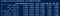One Way ANOVA + Games Howell Test

In summary we have highlighted the issues with naively applying Sharpe ratios to estimate risk adjusted fund performance and developed a new ratio called the Intrinsic Return which can be readily estimated using ARMA GARCH model in R. We show that this statistic when used jointly with the Sharpe ratio provides a statistically significant improvement in our screening procedure for future returns by introducing statistically correct confidence intervals.

Code for Auto ARMA GARCH Fitting: Github Gist.

Code for Games Howell Test: Github Gist